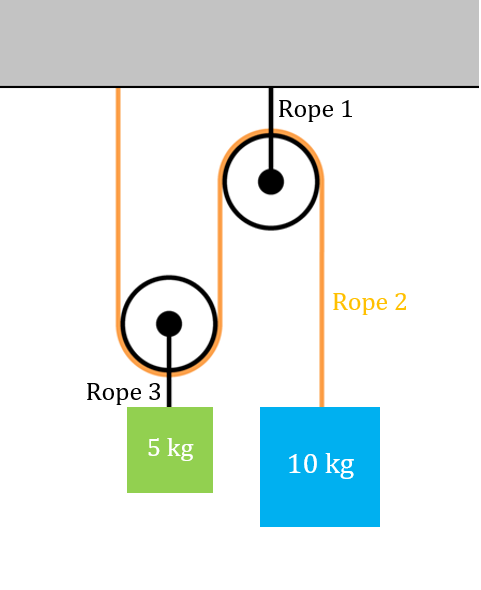# A constrained pulley!• The system is initially kept at rest, but is now released.
• Find the $\green{\underline{\text{acceleration}}}$ of the $\green{\underline{5 \text{kg}}}$ block in $\red{\underline{\text{ms}^{-2}}}$. Let it be $\green{a}$.
• Find the $\orange{\underline{\text{tension}}}$ of the $\orange{\underline{\text{Rope 2}}}$ block in $\red{\underline{\text{newtons}}}$. Let it be $\orange{T}$.
• Enter your answer as the $\green{|a|}+\orange{|T|}$, rounded up to two decimal places.

#### $\underline{\red{\text{Details and assumptions.}}}$

• All strings and pulleys are ideal.
• Ignore air resistance.
• Assume the ceiling is rigid.
• Take $\purple{\underline{\text{Acceleration due to gravity = 10 ms}^{-2}}}$

$\boxed{\large{\text{Difficulty: }\color{#D61F06}{\text{Small, but pointy}}} \LARGE{\red{\dagger \dagger } \dagger \dagger \dagger}}$

This problem is original.

×